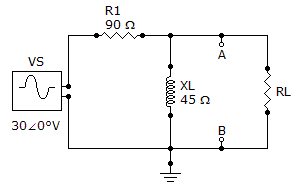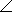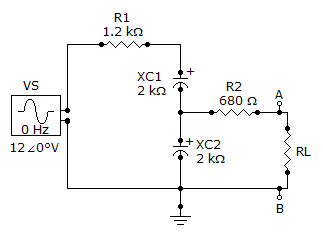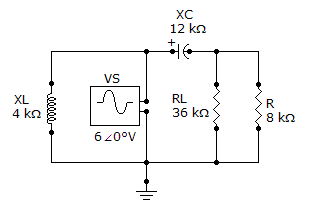# Electrical Engineering - Circuit Theorems in AC Analysis

### Exercise :: Circuit Theorems in AC Analysis - General Questions

6.

Determine VTH for the circuit external to RL in the given figure.A. 13563.4° V B. 13.563.4° V C. 13.50° V D. 1350° V

Explanation:

No answer description available for this question. Let us discuss.

7.

Referring to the given figure, determine ZTH as seen by RL if R1 is changed to 3.3 k.A. 1488–70.7°B. 3859–31.2°C. 5180–50.5°D. 1828–50.2°Explanation:

No answer description available for this question. Let us discuss.

8.

The two basic components of a Thevenin equivalent ac circuit are

 A. the equivalent voltage source and the equivalent series impedance B. the equivalent voltage source and the equivalent series resistance C. the equivalent voltage source and the equivalent parallel impedance D. the equivalent voltage source and the equivalent parallel resistance

Explanation:

No answer description available for this question. Let us discuss.

9.

Referring to the given circuit, find ZTH if VS is 180° V.A. 9.82–51.3° kB. 9.38–51.3° kC. 180–38.3° kD. 19.2–38.3° kExplanation:

No answer description available for this question. Let us discuss.

10.

In applying the superposition theorem,

 A. the sources are considered one at a time with all others replaced by their internal impedance B. all sources are considered independently C. all sources are considered simultaneously D. the sources are considered one at a time with all others replaced by their internal resistance